# Transforms and Resampling¶

This notebook explains how to apply transforms to images, and how to perform image resampling.

In :
from __future__ import print_function
import SimpleITK as sitk
import numpy as np
%matplotlib inline
from matplotlib import pyplot as plt
from ipywidgets import interact, fixed

# Utility method that either downloads data from the Girder repository or


## Creating and Manipulating Transforms¶

A number of different spatial transforms are available in SimpleITK.

The simplest is the Identity Transform. This transform simply returns input points unaltered.

In :
dimension = 2

print('*Identity Transform*')
identity = sitk.Transform(dimension, sitk.sitkIdentity)
print('Dimension: ' + str(identity.GetDimension()))

# Points are always defined in physical space
point = (1.0, 1.0)
def transform_point(transform, point):
transformed_point = transform.TransformPoint(point)
print('Point ' + str(point) + ' transformed is ' + str(transformed_point))

transform_point(identity, point)

*Identity Transform*
Dimension: 2
Point (1.0, 1.0) transformed is (1.0, 1.0)


Transform are defined by two sets of parameters, the Parameters and FixedParameters. FixedParameters are not changed during the optimization process when performing registration. For the TranslationTransform, the Parameters are the values of the translation Offset.

In :
print('*Translation Transform*')
translation = sitk.TranslationTransform(dimension)

print('Parameters: ' + str(translation.GetParameters()))
print('Offset:     ' + str(translation.GetOffset()))
print('FixedParameters: ' + str(translation.GetFixedParameters()))
transform_point(translation, point)

print('')
translation.SetParameters((3.1, 4.4))
print('Parameters: ' + str(translation.GetParameters()))
transform_point(translation, point)

*Translation Transform*
Parameters: (0.0, 0.0)
Offset:     (0.0, 0.0)
FixedParameters: ()
Point (1.0, 1.0) transformed is (1.0, 1.0)

Parameters: (3.1, 4.4)
Point (1.0, 1.0) transformed is (4.1, 5.4)


The affine transform is capable of representing translations, rotations, shearing, and scaling.

In :
print('*Affine Transform*')
affine = sitk.AffineTransform(dimension)

print('Parameters: ' + str(affine.GetParameters()))
print('FixedParameters: ' + str(affine.GetFixedParameters()))
transform_point(affine, point)

print('')
affine.SetTranslation((3.1, 4.4))
print('Parameters: ' + str(affine.GetParameters()))
transform_point(affine, point)

*Affine Transform*
Parameters: (1.0, 0.0, 0.0, 1.0, 0.0, 0.0)
FixedParameters: (0.0, 0.0)
Point (1.0, 1.0) transformed is (1.0, 1.0)

Parameters: (1.0, 0.0, 0.0, 1.0, 3.1, 4.4)
Point (1.0, 1.0) transformed is (4.1, 5.4)


A number of other transforms exist to represent non-affine deformations, well-behaved rotation in 3D, etc. See the Transforms tutorial for more information.

## Applying Transforms to Images¶

Create a function to display the images that is aware of image spacing.

In :
def myshow(img, title=None, margin=0.05, dpi=80):
nda = sitk.GetArrayViewFromImage(img)
spacing = img.GetSpacing()

ysize = nda.shape
xsize = nda.shape

figsize = (1 + margin) * ysize / dpi, (1 + margin) * xsize / dpi

fig = plt.figure(title, figsize=figsize, dpi=dpi)
ax = fig.add_axes([margin, margin, 1 - 2*margin, 1 - 2*margin])

extent = (0, xsize*spacing, 0, ysize*spacing)

t = ax.imshow(nda,
extent=extent,
interpolation='hamming',
cmap='gray',
origin='lower')

if(title):
plt.title(title)


Create a grid image.

In :
grid = sitk.GridSource(outputPixelType=sitk.sitkUInt16,
size=(250, 250),
sigma=(0.5, 0.5),
gridSpacing=(5.0, 5.0),
gridOffset=(0.0, 0.0),
spacing=(0.2,0.2))
myshow(grid, 'Grid Input')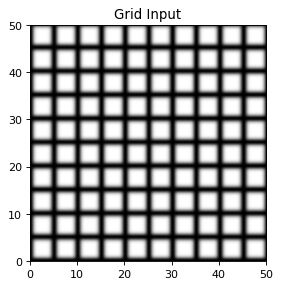To apply the transform, a resampling operation is required.

In :
def resample(image, transform):
# Output image Origin, Spacing, Size, Direction are taken from the reference
# image in this call to Resample
reference_image = image
interpolator = sitk.sitkCosineWindowedSinc
default_value = 100.0
return sitk.Resample(image, reference_image, transform,
interpolator, default_value)

translation.SetOffset((3.1, 4.6))
transform_point(translation, point)
resampled = resample(grid, translation)
myshow(resampled, 'Resampled Translation')

Point (1.0, 1.0) transformed is (4.1, 5.6)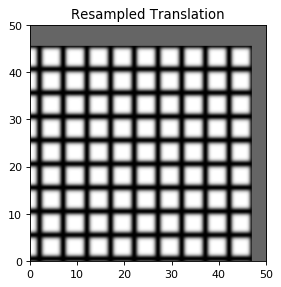What happened? The translation is positive in both directions. Why does the output image move down and to the left? It important to keep in mind that a transform in a resampling operation defines the transform from the output space to the input space.

In :
translation.SetOffset(-1*np.array(translation.GetParameters()))
transform_point(translation, point)
resampled = resample(grid, translation)
myshow(resampled, 'Inverse Resampled')

Point (1.0, 1.0) transformed is (-2.1, -3.5999999999999996)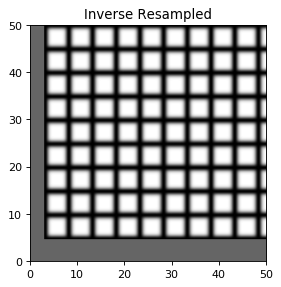An affine (line preserving) transformation, can perform translation:

In :
def affine_translate(transform, x_translation=3.1, y_translation=4.6):
new_transform = sitk.AffineTransform(transform)
new_transform.SetTranslation((x_translation, y_translation))
resampled = resample(grid, new_transform)
myshow(resampled, 'Translated')
return new_transform

affine = sitk.AffineTransform(dimension)

interact(affine_translate, transform=fixed(affine), x_translation=(-5.0, 5.0), y_translation=(-5.0, 5.0))

Out:
<function __main__.affine_translate(transform, x_translation=3.1, y_translation=4.6)>

or scaling:

In :
def affine_scale(transform, x_scale=3.0, y_scale=0.7):
new_transform = sitk.AffineTransform(transform)
matrix = np.array(transform.GetMatrix()).reshape((dimension,dimension))
matrix[0,0] = x_scale
matrix[1,1] = y_scale
new_transform.SetMatrix(matrix.ravel())
resampled = resample(grid, new_transform)
myshow(resampled, 'Scaled')
print(matrix)
return new_transform

affine = sitk.AffineTransform(dimension)

interact(affine_scale, transform=fixed(affine), x_scale=(0.2, 5.0), y_scale=(0.2, 5.0))

Out:
<function __main__.affine_scale(transform, x_scale=3.0, y_scale=0.7)>

or rotation:

In :
def affine_rotate(transform, degrees=15.0):
parameters = np.array(transform.GetParameters())
new_transform = sitk.AffineTransform(transform)
matrix = np.array(transform.GetMatrix()).reshape((dimension,dimension))
radians = -np.pi * degrees / 180.
new_matrix = np.dot(rotation, matrix)
new_transform.SetMatrix(new_matrix.ravel())
resampled = resample(grid, new_transform)
print(new_matrix)
myshow(resampled, 'Rotated')
return new_transform

affine = sitk.AffineTransform(dimension)

interact(affine_rotate, transform=fixed(affine), degrees=(-90.0, 90.0))

Out:
<function __main__.affine_rotate(transform, degrees=15.0)>

or shearing:

In :
def affine_shear(transform, x_shear=0.3, y_shear=0.1):
new_transform = sitk.AffineTransform(transform)
matrix = np.array(transform.GetMatrix()).reshape((dimension,dimension))
matrix[0,1] = -x_shear
matrix[1,0] = -y_shear
new_transform.SetMatrix(matrix.ravel())
resampled = resample(grid, new_transform)
myshow(resampled, 'Sheared')
print(matrix)
return new_transform

affine = sitk.AffineTransform(dimension)

interact(affine_shear, transform=fixed(affine), x_shear=(0.1, 2.0), y_shear=(0.1, 2.0))

Out:
<function __main__.affine_shear(transform, x_shear=0.3, y_shear=0.1)>

## Composite Transform¶

It is possible to compose multiple transform together into a single transform object. With a composite transform, multiple resampling operations are prevented, so interpolation errors are not accumulated. For example, an affine transformation that consists of a translation and rotation,

In :
translate = (8.0, 16.0)
rotate = 20.0

affine = sitk.AffineTransform(dimension)
affine = affine_translate(affine, translate, translate)
affine = affine_rotate(affine, rotate)

resampled = resample(grid, affine)
myshow(resampled, 'Single Transform')

[[ 0.93969262  0.34202014]
[-0.34202014  0.93969262]]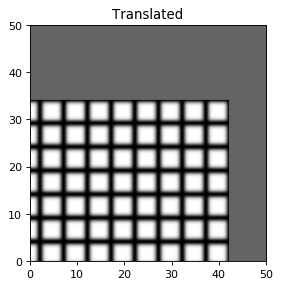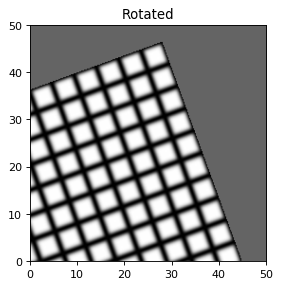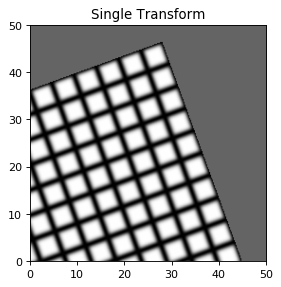can also be represented with two Transform objects applied in sequence with a Composite Transform,

In :
composite = sitk.Transform(dimension, sitk.sitkComposite)
translation = sitk.TranslationTransform(dimension)
translation.SetOffset(-1*np.array(translate))
affine = sitk.AffineTransform(dimension)
affine = affine_rotate(affine, rotate)

composite = sitk.Transform(dimension, sitk.sitkComposite)

resampled = resample(grid, composite)
myshow(resampled, 'Two Transforms')

[[ 0.93969262  0.34202014]
[-0.34202014  0.93969262]]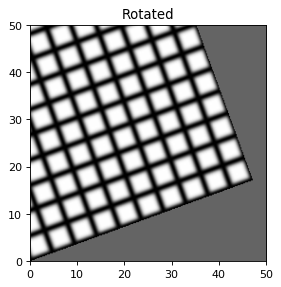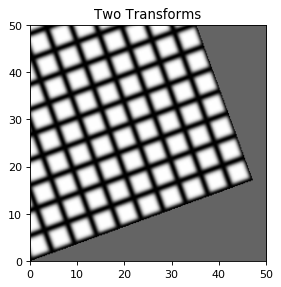Beware, tranforms are non-commutative -- order matters!

In :
composite = sitk.Transform(dimension, sitk.sitkComposite)

resampled = resample(grid, composite)
myshow(resampled, 'Composite transform in reverse order')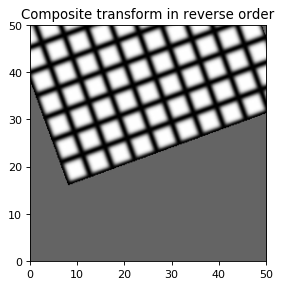## Resampling¶Resampling as the verb implies is the action of sampling an image, which itself is a sampling of an original continuous signal.

Generally speaking, resampling in SimpleITK involves four components:

1. Image - the image we resample, given in coordinate system $m$.
2. Resampling grid - a regular grid of points given in coordinate system $f$ which will be mapped to coordinate system $m$.
3. Transformation $T_f^m$ - maps points from coordinate system $f$ to coordinate system $m$, $^mp = T_f^m(^fp)$.
4. Interpolator - method for obtaining the intensity values at arbitrary points in coordinate system $m$ from the values of the points defined by the Image.

While SimpleITK provides a large number of interpolation methods, the two most commonly used are sitkLinear and sitkNearestNeighbor. The former is used for most interpolation tasks, a compromise between accuracy and computational efficiency. The later is used to interpolate labeled images representing a segmentation, it is the only interpolation approach which will not introduce new labels into the result.

SimpleITK's procedural API provides three methods for performing resampling, with the difference being the way you specify the resampling grid:

1. Resample(const Image &image1, Transform transform, InterpolatorEnum interpolator, double defaultPixelValue, PixelIDValueEnum outputPixelType)
2. Resample(const Image &image1, const Image &referenceImage, Transform transform, InterpolatorEnum interpolator, double defaultPixelValue, PixelIDValueEnum outputPixelType)
3. Resample(const Image &image1, std::vector< uint32_t > size, Transform transform, InterpolatorEnum interpolator, std::vector< double > outputOrigin, std::vector< double > outputSpacing, std::vector< double > outputDirection, double defaultPixelValue, PixelIDValueEnum outputPixelType)
In :
def resample_display(image, euler2d_transform, tx, ty, theta):
euler2d_transform.SetTranslation((tx, ty))
euler2d_transform.SetAngle(theta)

resampled_image = sitk.Resample(image, euler2d_transform)
plt.imshow(sitk.GetArrayFromImage(resampled_image))
plt.axis('off')
plt.show()

euler2d = sitk.Euler2DTransform()
# Why do we set the center?
euler2d.SetCenter(logo.TransformContinuousIndexToPhysicalPoint(np.array(logo.GetSize())/2.0))
interact(resample_display, image=fixed(logo), euler2d_transform=fixed(euler2d), tx=(-128.0, 128.0,2.5), ty=(-64.0, 64.0), theta=(-np.pi/4.0,np.pi/4.0));

Fetching SimpleITK.jpg


### Common Errors¶

It is not uncommon to end up with an empty (all black) image after resampling. This is due to:

1. Using wrong settings for the resampling grid, not too common, but does happen.
2. Using the inverse of the transformation $T_f^m$. This is a relatively common error, which is readily addressed by invoking the transformations GetInverse method.

### Defining the Resampling Grid¶

In the example above we arbitrarily used the original image grid as the resampling grid. As a result, for many of the transformations the resulting image contained black pixels, pixels which were mapped outside the spatial domain of the original image and a partial view of the original image.

If we want the resulting image to contain all of the original image no matter the transformation, we will need to define the resampling grid using our knowledge of the original image's spatial domain and the inverse of the given transformation.

Computing the bounds of the resampling grid when dealing with an affine transformation is straightforward. An affine transformation preserves convexity with extreme points mapped to extreme points. Thus we only need to apply the inverse transformation to the corners of the original image to obtain the bounds of the resampling grid.

Computing the bounds of the resampling grid when dealing with a BSplineTransform or DisplacementFieldTransform is more involved as we are not guaranteed that extreme points are mapped to extreme points. This requires that we apply the inverse transformation to all points in the original image to obtain the bounds of the resampling grid.

In :
euler2d = sitk.Euler2DTransform()
# Why do we set the center?
euler2d.SetCenter(logo.TransformContinuousIndexToPhysicalPoint(np.array(logo.GetSize())/2.0))

tx = 64
ty = 32
euler2d.SetTranslation((tx, ty))

extreme_points = [logo.TransformIndexToPhysicalPoint((0,0)),
logo.TransformIndexToPhysicalPoint((logo.GetWidth(),0)),
logo.TransformIndexToPhysicalPoint((logo.GetWidth(),logo.GetHeight())),
logo.TransformIndexToPhysicalPoint((0,logo.GetHeight()))]
inv_euler2d = euler2d.GetInverse()

extreme_points_transformed = [inv_euler2d.TransformPoint(pnt) for pnt in extreme_points]
min_x = min(extreme_points_transformed)
min_y = min(extreme_points_transformed, key=lambda p: p)
max_x = max(extreme_points_transformed)
max_y = max(extreme_points_transformed, key=lambda p: p)

# Use the original spacing (arbitrary decision).
output_spacing = logo.GetSpacing()
# Identity cosine matrix (arbitrary decision).
output_direction = [1.0, 0.0, 0.0, 1.0]
# Minimal x,y coordinates are the new origin.
output_origin = [min_x, min_y]
# Compute grid size based on the physical size and spacing.
output_size = [int((max_x-min_x)/output_spacing), int((max_y-min_y)/output_spacing)]

resampled_image = sitk.Resample(logo, output_size, euler2d, sitk.sitkLinear, output_origin, output_spacing, output_direction)
plt.imshow(sitk.GetArrayViewFromImage(resampled_image))
plt.axis('off')
plt.show()Are you puzzled by the result? Is the output just a copy of the input? Add a rotation to the code above and see what happens (euler2d.SetAngle(0.79)).

### Resampling at a set of locations¶

In some cases you may be interested in obtaining the intensity values at a set of points (e.g. coloring the vertices of a mesh model segmented from an image).

The code below generates a random point set in the image and resamples the intensity values at these locations. It is written so that it works for all image-dimensions and types (scalar or vector pixels).

In :
img = logo

# Generate random samples inside the image, we will obtain the intensity/color values at these points.
num_samples = 10
physical_points = []
for pnt in zip(*[list(np.random.random(num_samples)*sz) for sz in img.GetSize()]):
physical_points.append(img.TransformContinuousIndexToPhysicalPoint(pnt))

# Create an image of size [num_samples,1...1], actual size is dependent on the image dimensionality. The pixel
# type is irrelevant, as the image is just defining the interpolation grid (sitkUInt8 has minimal memory footprint).
interp_grid_img = sitk.Image([num_samples] + *(img.GetDimension()-1), sitk.sitkUInt8)

# Define the displacement field transformation, maps the points in the interp_grid_img to the points in the actual
# image.
displacement_img = sitk.Image([num_samples] + *(img.GetDimension()-1), sitk.sitkVectorFloat64, img.GetDimension())
for i, pnt in enumerate(physical_points):
displacement_img[[i] + *(img.GetDimension()-1)] = np.array(pnt) - np.array(interp_grid_img.TransformIndexToPhysicalPoint([i] + *(img.GetDimension()-1)))

# Actually perform the resampling. The only relevant choice here is the interpolator. The default_output_pixel_value
# is set to 0.0, but the resampling should never use it because we expect all points to be inside the image and this
# value is only used if the point is outside the image extent.
interpolator_enum = sitk.sitkLinear
default_output_pixel_value = 0.0
output_pixel_type = sitk.sitkFloat32 if img.GetNumberOfComponentsPerPixel()==1 else sitk.sitkVectorFloat32
resampled_points = sitk.Resample(img, interp_grid_img, sitk.DisplacementFieldTransform(displacement_img),
interpolator_enum, default_output_pixel_value, output_pixel_type)

# Print the interpolated values per point
for i in range(resampled_points.GetWidth()):
print(str(physical_points[i]) + ': ' + str(resampled_points[[i] + *(img.GetDimension()-1)]) + '\n')

(31.979373973128354, 31.665540199030733): (248.3671417236328, 234.6564178466797, 206.66815185546875)

(110.22152719753338, 14.216197227312362): (255.0, 255.0, 255.0)

(113.1327139020932, 17.32004321736616): (253.2654266357422, 253.13272094726562, 254.44485473632812)

(125.55050116130072, 31.535179402668156): (45.02242660522461, 86.90109252929688, 168.87074279785156)

(122.05631183529644, 18.357515620495025): (201.74758911132812, 191.71141052246094, 198.1728973388672)

(130.40924540626676, 48.118765664156285): (255.0, 255.0, 255.0)

(197.34300068021432, 41.19593678155369): (255.0, 255.0, 255.0)

(107.34115235214041, 17.459751807254353): (255.0, 255.0, 255.0)

(105.3285249869318, 6.585967746278851): (255.0, 255.0, 255.0)

(119.6239842407568, 17.571440050113576): (161.7855224609375, 163.1717071533203, 159.46429443359375)



## Homework: creating a color mesh¶

You will now use the code for resampling at arbitrary locations to create a colored mesh.

Using the color image of the visible human head [img = sitk.ReadImage(fdata('vm_head_rgb.mha'))]:

1. Implement the marching cubes algorithm to obtain the set of triangles corresponding to the iso-surface of structures of interest (skin, white matter,...). The original paper is available from the authors here.
2. Find the color associated with each of the triangle vertices using the code above.
3. Save the data using the ASCII version of the PLY), Polygon File Format (a.k.a. Stanford Triangle Format).
4. Use meshlab to view your creation.